# Brushed DC Motor Theory

(Difference between revisions)

### How does a motor work?

Let's consider a permanent magnet brushed motor. The piece connected to the ground is called the stator and the piece connected to the output shaft is called the rotor. The inputs of the motor are connected to 2 wires and by applying a voltage across them, the motor turns.

The torque of a motor is generated by a current carrying conductor in a magnetic field. The right hand rule states that if you point your right hand fingers along the direction of current, I, and curl them towards the direction of the magnetic flux, B, the direction of force is along the thumb.

Now, imagine a loop of wire with some resistance is inserted between the two permanent magnets. The following diagrams show how the motor turns:

 File:Motor2.jpg Diagram showing how the motor works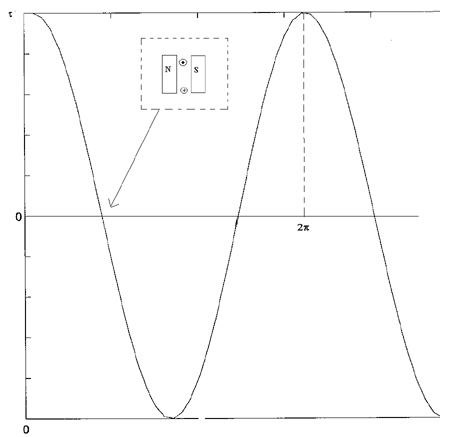You might be able to notice that the direction of rotation is changing every half cycle. To keep it rotating in the same direction, we have to switch the current direction. The process of switching current is called commutation. To switch the direction of curent, we have to use brushes and commutators. Commutation can also be done electronically (Brushless motors) and a brushless motor usually has a longer life. The following diagram shows how brushes and commutators work.

We could also have several commutators and loops. The total torque generated is the sum of all the torques from each of the loops added.

 File:Brush.jpg Motor with several commutators and loops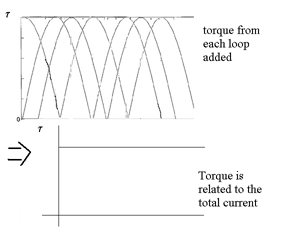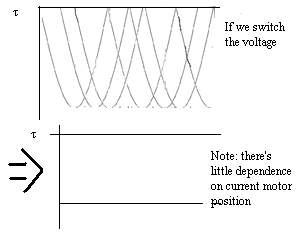### Equations

So, the torque is proportional to the current through the windings,

τ = kI,

where τ is the torque, I is the current, and k is a constant. The wire coils have both a resistance, R, and an inductance, L. When the motor is turning, the current is switching, causing a voltage,$V = L \begin{matrix}\frac{dI}{dt}\end{matrix}$

This voltage is known as the back-emf(electromotive force), ε. If the angular velocuty of the motor is ω, then

ε = kω,

like a generator. This voltage, ε, is working against the voltage we apply across the terminals, and so,

(Vkω) = IR,

where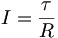$I = \frac{\tau}{R}$

which implies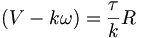$(V-k\omega) = \frac{\tau}{k}R$.

The maximum or stall torque is the torque at which ω = 0 or$\tau_{stall} = \frac{kV}{R}$,

and the stall or starting current,$I_{start} = \frac{V}{R}$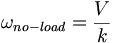$\omega_{no-load} = \frac{V}{k}$,

is the maximum speed the motor can run. Given a constant voltage, the motor will settle at a constant speed, just like a terminal velocity. If we plot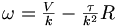$\omega = \begin{matrix}\frac{V}{k}\end{matrix} - \begin{matrix}\frac{\tau}{k^2}\end{matrix}R$,

we can get the speed-torque curve:

### Units

Here are the different units for the torque, current and voltage

 Torque τ Nm (=kgm/s^2*m), kgfm(=9.8 times Nm), gfcm, mNm, etc Current I Amperes(Amps), mA Voltage V Volts Mechanical Power τω 1 Nm/sec = 1 watt Electrical Power VI 1 volt*amp = 1 watt